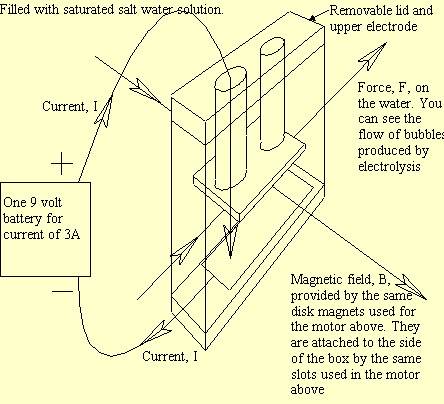This section contains pictures and descriptions of the MHD motor, static display and boat which I used in my presentation.

• Firstly, you need to see how many batteries you need to carry on the boat. By experimentation and calculation it was found that the force produced by a current of 3A, supplied by a 9 volt battery, would do nicely. Is 9 volts enough to produce this current in a saturated salt water solution?
• Use R = d/(A) where d is the distance the current travels
• A is the cross sectional area of the material in which the current is traveling

is the conductivity of the material

In this case the material is a saturated salt water solution and:

• d = 0.04 m is the distance between the electrodes
• A = 0.0008 m2 is the area of an electrode = 22.6 (m)-1 for saturated salt water at 20 deg. Celsius
• Carrying the calculation through to get R = 2.21 . Now use Ohms Law: V = IR where I = 3A (short-circuit current of a 9 volt battery) V = 6.64 volts So a 9 volt battery should do the job.
• If the water is not saturated, which was the case with my project, you might want to put some more batteries in series. (I had 3 in series; on the heave side)
• # The Motor# The Static DisplayThe Boat was made out of a large piece of plastic Styrofoam and looked a bit like a barge.

The motor was attached to the bottom by the copper rods (see above). The batteries and switching mechanism (to switch the polarity of the electrodes) were contained in a waterproof Tupperware container mounted on top of the barge.

(Sorry, no picture due to technical difficulties. You're just going to have to imagine it.)